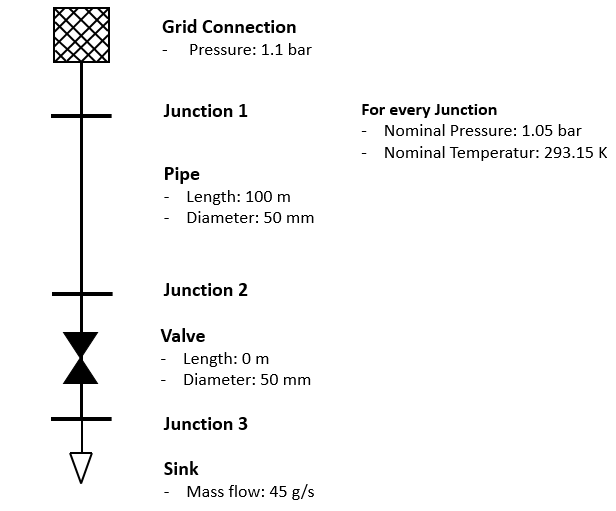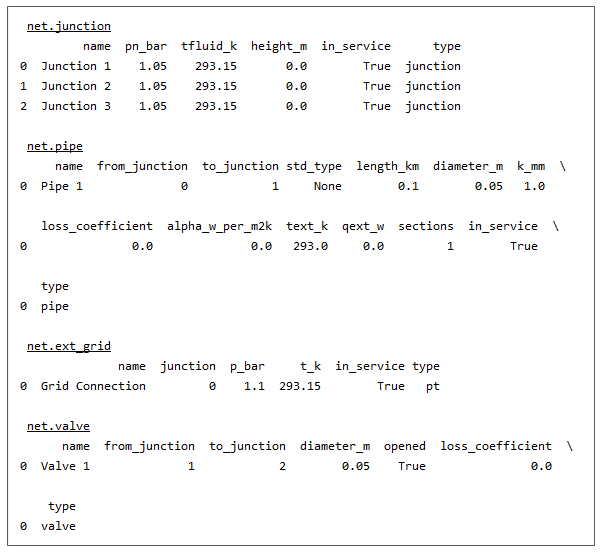Getting Started

Installing pandapower

For the installation of pandapipes it is necessary to install pandapower first. For the installation please follow the detailed instructions which can be found on the pandapower website.

Installing pandapipes

Through pip

The easiest way to install pandapipes is through pip:

1. Open a command prompt (e.g. start –> cmd on windows systems)

2. Install pandapipes by running:

pip install pandapipes

Without pip

If you don’t have internet access on your system or don’t want to use pip for some other reason, pandapipes can also be installed without using pip:

2. Open a command prompt (e.g. start –> cmd on windows systems) and navigate to the folder that contains the setup.py file with the command cd <folder> :

cd %path_to_pandapipes%\pandapipes-x.x.x\

3. Install pandapipes by running :

python setup.py install

Development Version

To install the latest development version of pandapipes from github, simply follow these steps:

2. Open a git shell and navigate to the directory where you want to keep your pandapipes files.

3. Run the following git command:

git clone https://github.com/e2nIEE/pandapipes.git

4. Navigate inside the repository and check out the develop branch:

cd pandapipes
git checkout develop

5. Open a command prompt (cmd or anaconda command prompt) and navigate to the folder where the pandapipes files are located. Run:

pip install -e .

This registers your local pandapipes installation with pip.

A first basic way to test your installation is to import all pandapower submodules to see if all dependencies are available:

import pandapipes
import pandapipes.plotting
import pandapipes.timeseries
import pandapipes.networks
import pandapipes.io
import pandapipes.control
import pandapipes.component_models

If you want to be really sure that everything works fine, run the pandapower test suite:

1. Install pytest if it is not yet installed on your system:

pip install pytest

2. Run the pandapower test suite:

import pandapipes.test
pandapipes.test.run_tests()

If everything is installed correctly, all tests should pass or xfail (expected to fail).

A short introduction

A network in pandapipes is represented in a pandapipesNet object, which is a collection of pandas Dataframes. Each dataframe in a pandapipesNet contains the information about one of pandapipes’ component types, such as pipe, junction, sink, etc.. Each row represents an element of the respective type.

We consider the following simple example network as a minimal example:Creating a network

The above network can be created in pandapipes as follows:

import pandapipes as pp
#create empty net
net = pp.create_empty_network(fluid="lgas")

#create junctions
j1 = pp.create_junction(net, pn_bar=1.05, tfluid_k=293.15, name="Junction 1")
j2 = pp.create_junction(net, pn_bar=1.05, tfluid_k=293.15, name="Junction 2")
j3 = pp.create_junction(net, pn_bar=1.05, tfluid_k=293.15, name="Junction 3")

#create junction elements
ext_grid = pp.create_ext_grid(net, junction=j1, p_bar=1.1, t_k=293.15, name="Grid Connection")
sink = pp.create_sink(net, junction=j3, mdot_kg_per_s=0.045, name="Sink")

#create branch elements
pipe = pp.create_pipe_from_parameters(net, from_junction=j1, to_junction=j2, length_km=0.1, diameter_m=0.05, name="Pipe")
valve = pp.create_valve(net, from_junction=j2, to_junction=j3, diameter_m=0.05, opened=True, name="Valve")

The pandapipes representation now looks like this:Running a Pipe Flow

A pipeflow can be carried out with the pipeflow function:

pp.pipeflow(net)

When a pipeflow is run, pandapipes combines the information of all element tables and calculates the current state of the network. Afterwards, results are written into the result tables.

This minimal example is also available as a jupyter notebook.

Interactive Tutorials

There are jupyter notebook tutorials on different functionalities of pandapipes. They can be viewed as static tutorials on GitHub or as interactive tutorials via Binder. Interactive tutorials take a moment to load.

Pandapipes provides different calculation modes and, depending on the task you want to apply pandapipes to, you will not have to do all tutorials. Read the following descriptions in order to determine the tutorials which are important for you.

The tutorials linked in this section describe how pandapipes can be used to calculate the pressure and velocity distribution in a network in case of static boundary conditions. Examples are given for compressible and incompressible media. Because every other calculation includes the calculation of network hydraulics, this section is a good place to begin:

Some tutorials on the calculation of network hydraulics are also available in German:

Static thermal calculations:

If not only network hydraulics are of interest, but also temperature values along the pipes and nodes have to be known, you can have a look at the following tutorial:

In the special case of a district heating grid, the fluid flows in a closed loop. Heat is extracted by the consumers of the district heating network, which can be modeled in pandapipes with heat exchangers. To model flow in a closed loop, pandapipes provides a special kind of pump, which is introduced in this tutorial:

Quasistatic timeseries calculations:

With pandapipes, quasistatic processes can be modeled. For example, a timeseries describing values of an extracted mass flow over several time steps can be read. For each time step value, a pandapipes calculation is done automatically. A tutorial describing the basic setup of these calculations can be found here:

Coupled pandapower-pandapipes simulations:

This tutorial shows how to use pandapipes in combination with pandapower to solve a time-dependent sector coupling application. Also, a German version is available:

• Introduction to coupled simulation of power and gas networks (multinet) (static / interactive)
• German version of tutorial for coupled simulations (static / interactive)

Miscellaneous:

In this last section, you can find tutorials on auxiliary functions which are useful for all pandapipes users: